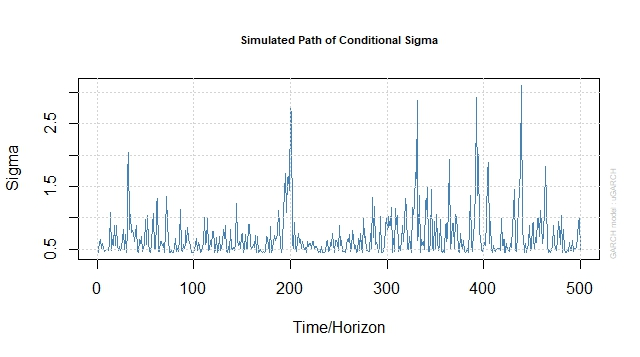Want to share your content on R-bloggers? click here if you have a blog, or here if you don't.This is the second part of the series on volatility modelling. For other parts of the series follow the tag volatility.

In this exercise set we will use the `dmbp` dataset from part-1 and extend our analysis to GARCH (Generalized Autoregressive Conditional Heteroscedasticity) models.

Answers to the exercises are available here.

Exercise 1
Load the `rugarch` package and the `dmbp` dataset (Bollerslev, T. and Ghysels, E. 1996, Periodic Autoregressive Conditional Heteroscedasticity, Journal
of Business and Economic Statistics, 14, 139–151). This dataset has daily logarithmic nominal returns for Deutsche-mark / Pound. There is also a dummy variable to indicate non-trading days.
Define the daily return as a time series variable.

Exercise 2
We will first simulate and analyze a GARCH process. Use the `ugarchspec` function to define an GARCH(1,1) process. The return has a simple mean specification with mean=0. The variance follows as AR-1 process with constant=0.2, AR-1 coefficient = 0.2 and MA-1 coefficient = 0.6.

Exercise 3
Simulate the defined GARCH process for 500 time periods. Exercises 4 to 6 use this simulated data.

Exercise 4
Plot the returns vs time and note the apparent unpredictability. Plot the path of conditional sigma vs time and note that there is some persistence over time.

Learn more about Model Evaluation in the online course Regression Machine Learning with R. In this course you will learn how to:

• Avoid model over-fitting using cross-validation for optimal parameter selection
• Explore maximum margin methods such as best penalty of error term support vector machines with linear and non-linear kernels.
• And much more

Exercise 5
Plot the ACF of returns and squared returns. Note that there is no auto-correlation between returns but squared returns have significant first degree autocorrelation.

Exercise 6
Test for ARCH effects using the Ljung Box test for the simulated data.

Exercise 7
We will now use the currency returns data. Use the `ugarchfit` function to estimate a GARCH(1,1) model for the data. The return has a simple mean specification with mean=0.

Exercise 8
Plot the fit diagnostics graphs.

Exercise 9
Fit an AR(1)-GARCH(1,1) model to the data.

Exercise 10
Plot the fit diagnostics graphs for the new model.